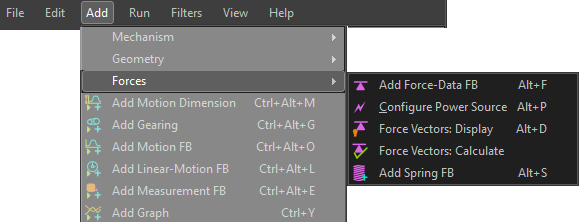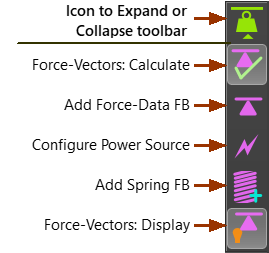﻿ MechDesigner Reference & User Interface > 2.2 Mechanism-Editor: > Force elements

# Force elements

## Forces elements

Use Force elements to:

Calculate Forces, Show Force Vectors (all Force types).

Configure the Power-Source.

Add a Force between two anchor-point.

Measure the force that acts on an element.On-line Tutorial: Tutorial 13: Forces Introduction